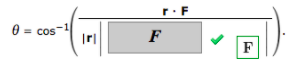# Problem: A wrench 40 cm long lies along the positive y-axis and grips a bolt at the origin. A force is applied in the direction (0, 4, -3) at the end of the wrench. Find the magnitude of the force needed to supply 90 N-m of torque to the bolt.In order to find |F|, we will have to find the angle between F and r. We can use the equationUsing the equation above, we see that the angle is as follows. (Round your answer to one decimal place.)

###### FREE Expert Solution

Torque formula is:

$\overline{){\mathbf{\tau }}{\mathbf{=}}{\mathbf{r}}{\mathbf{F}}{\mathbf{s}}{\mathbf{i}}{\mathbf{n}}{\mathbf{\theta }}}$

In this problem, we'll compute the angle between r and F from the equation:

$\overline{){\mathbf{\theta }}{\mathbf{=}}{\mathbf{c}}{\mathbf{o}}{{\mathbf{s}}}^{\mathbf{-}\mathbf{1}}{\mathbf{\left(}}\frac{\mathbf{r}\mathbf{.}\mathbf{F}}{\mathbf{|}\mathbf{r}\mathbf{|}\mathbf{|}\mathbf{F}\mathbf{|}}{\mathbf{\right)}}}$

We'll also use vector magnitude:

$\overline{){\mathbf{|}}{\mathbf{r}}{\mathbf{|}}{\mathbf{=}}\sqrt{{\mathbf{x}}^{\mathbf{2}}\mathbf{+}{\mathbf{y}}^{\mathbf{2}}\mathbf{+}{\mathbf{z}}^{\mathbf{2}}}}$

98% (356 ratings)###### Problem Details

A wrench 40 cm long lies along the positive y-axis and grips a bolt at the origin. A force is applied in the direction (0, 4, -3) at the end of the wrench. Find the magnitude of the force needed to supply 90 N-m of torque to the bolt.

In order to find |F|, we will have to find the angle between F and r. We can use the equationUsing the equation above, we see that the angle is as follows. (Round your answer to one decimal place.)